Mode - Measures of Central Tendency, Business Mathematics & Statistics

# Mode - Measures of Central Tendency, Business Mathematics & Statistics - Business Mathematics and Statistics - B Com

MODE Definition :
Mode is the value of the variate which occurs most frequently. It represents the most frequent value of a series. In other words Mode is the value of the variable which has the highest frequency. When one speak of the ‘average student’, we generally mean the modal wage, the modal student. If we say that the modal wages obtained by workers in a factory are ` 70, we mean that the largest number of workers get the same amount. As high as ` 100 and as low as ` 50 as wages are much less frequented and they are non-modal.

Calculation. Mode cannot be determined in a series of individual observations unless it is converted to a discrete series (or continuous series). In a discrete series the value of the variate having the maximum frequency is the modal class. However, the exact location of mode is done by interpolation formula like median. Location of modal value in case of discrete series is possible if there is concentration of items at on point. If again there are two or more values having same maximum frequencies, (i.e. more concentration), it becomes difficult to determine mode. Such items are known as bimodal, tri-modal or multi-modal accordingly as the items concentrate at 2, 3 or more values.

(A) For. Individual Observations

The individual observations are to be first converted to discrete series (if possible). Then the variate having the maximum will be the mode. Example 34 : Calculate mode from the data (given) :

(Marks) : 10, 14, 24, 27, 24, 12, 11, 17.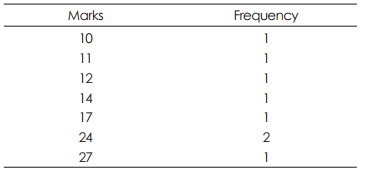(Individual observation are converted into a discrete series) Here marks 24 occurs maximum number of times, i.e. 2. Hence the modal marks is 24, or mode = 24 marks.

Alternatively : Arranging the numbers : 10, 11, 12, 14, 17, (24, 24) 27. Now 24 occurs maximum number of times, i.e. 2. ∴ Mode = 24 marks. [Note. When there are two or more values having the same maximum frequency, then mode is ill-defined. Such a sense is known as bimodal or multi-modal as the case may be.]

Example 35 : Compute mode from the following data. Marks obtained : 24, 14, 20, 17, 20, 14.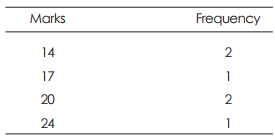[Here 14 occurs 2 times (max.) and 20 occurs 2 times (max.)
∴ mode is ill-defined.]

(B) For Simple Frequency Distribution Discrete Series.
To Find the mode from the following Table :

Height (in inches)               No. of Persons

57                                         3
59                                         5
61                                         7
62                                       10
63                                       20
64                                      22
65                                      24
66                                       5
67                                       2
69                                       2

Frequencies given below, in column (1) are grouped by two’s in column (2) and (3) and then by three’s in columns (4), (5), and (6). The maximum frequency in each column is marked by Bold Type. We do not find any fixed point having maximum frequency but changes with the change of grouping. In the following table, the sizes of maximum frequency in respect of different columns are arranged.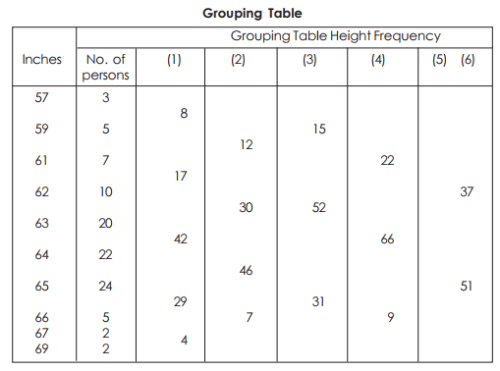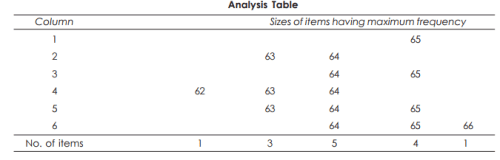From the above table, we find 64 is the size of the item which is most frequented. The mode is, therefore, located at 64. [Note. At glance from column (1) one might think that 65 is the mode since it contains maximum frequency. This impression is corrected by the process of grouping . So it is not advisable to locate the mode merely by inspection.]

(C) For continuous Series. By inspections or by preparing Grouping Table and Analysis Table, ascertain the modal class. Then to find the exact value of mode, apply the following formula.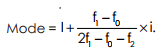Where, I = lower class-boundary of modal class
f1 = frequency of modal class.
f0 = frequency of the class preceding modal class.
f2 = frequency of the class succeeding the modal class.
i = size of the class- interval of modal class.

Note : the above formula may also be expressed as follows :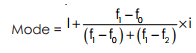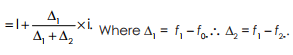Example 36 : Compute mode of the following distribution.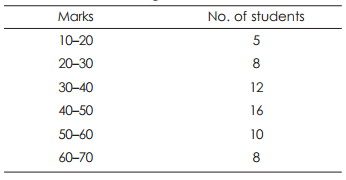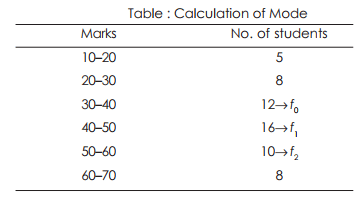From the table it is clear that the maximum frequency is 16th : modal class is (40–50) Here l = 40, f0 = 12, f1 = 16, f2 = 10 (marked in table), i = 10 (= 50 –40)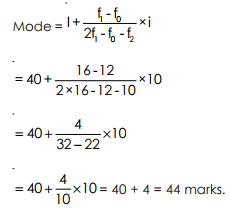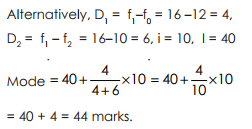Calculation of Mode From discrete group frequency distribution.

In such cases at first class boundaries are to be formed for applying formula.

Example 37: Compute mode from the following frequency distribution :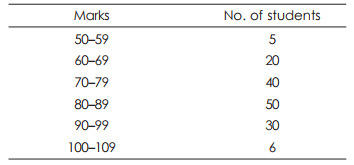The class intervals which are in discrete form are first converted into class boundaries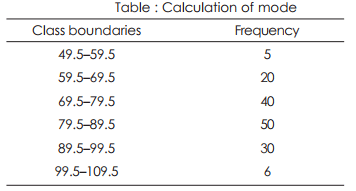Now modal class is (79.5 – 89.5), since this class has the highest frequency. Here l = 79.5, f0 = 40, f1 = 50, f2 = 30, i = 10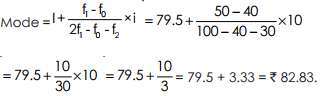Calculation of mode from cumulative frequency distribution :

Example 38 : From the following cumulative frequency distribution of marks of 22 students in Accountancy, calculate mode :

Marks              below 20          below 40       below 60        below 80          below 100
No. of students 3                         8                  17                   20                       22

Solution :

At first we are to transfer the above cumulative frequency distribution into a equal group frequency distribution and hence to calculate mode.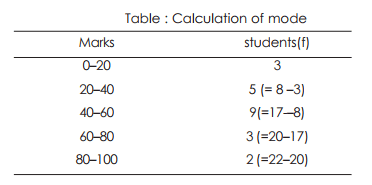Modal class is (40–60), as this class has highest frequency.
Here l = 40, f0 = 5, f1 = 9, f2 = 3, i = 20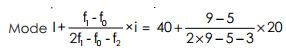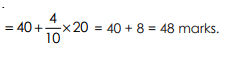Calculation of missing frequency :

Example 39 : Mode of the given distribution is 44, find the missing frequency

Marks                     10–20       20–30     30–40     40–50    50–60     60–70

No. of students           5              8           12              ––       10               8

Solution : Since mode is 44, so modal class is 40–50.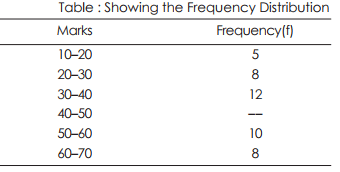let the missing frequency be f1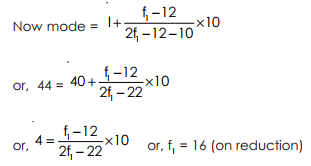Miscellaneous examples :

1. If two variates x and y are related by 2x = 3y – 1, and mean of y be 9 ; find the mean of x.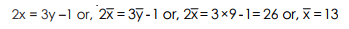2. If 2u = 5x is the relation between two variables x and u and harmonic mean of x is 0.4, find the harmonic mean of u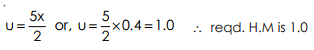3. The relation between two variables x and y is 3y – 2x + 5 = 0 and median of y is 40, find the median of x.

From 3y – 2x + 5 = 0 we get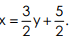As the median is located by position, so median of x is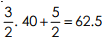4. Mode of the following frequency distribution is 24 and total frequency is 100. Find the values of f1 and f2 .

C.I :                  0 –10       10–20     20–30     30– 40     40–50
Frequency :      14                 f1         27           f2             15

Mode is 24, so modal class is (20–30). From the formula of mode we find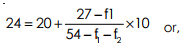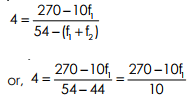14 + f1 + 27 + f2 + 15 = 100
or, f1 + f2 = 100 – 56 = 44 …….….(1)

or, 40 = 270 – 10f1 or, 10f1 = 230 or, f1 = 23. From (1) , f2 = 44 – 23 = 11 ∴ f1 = 23, f2 = 11

5. The following are the monthly salaries in rupees of 20 employees of a firm :
130     125    110     100      80      76     98   103  122     66
145      151    65        71    118    140    116    85   95      151

The firm gives bonuses of ` 10, 15, 20, 25 and 30 for individuals in the respective salary group : exceeding ` 60 but not excedding ` 80, exceeding ` 80 but not exceeding ` 100 and so on up to exceeding ` 140 but not exceeding ` 160. Find the average bonus paid per employee.

Solution: From the monthly salaries of the employees, we find the number of employees lying in the salary groups mentioned as follows :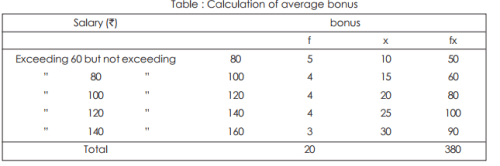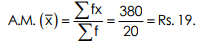Marks obtained by 30 students in History of a Test Examination 2012 of some school are as follows :
34        36      10     21     31     32    22    43    48     36
48        22       39    26      34     39   10    17     47    38
40        51       35     52      41     32   30    35     53    23

construct a frequency table with class intervals 10–19. 20–29 etc. Calculate the median and mode from the frequency distribution.

Solution: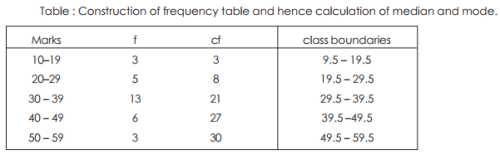Median = value of Nth/2 i.e., 30 2 i.e. 15th term
So median class is (29.5 – 39.5)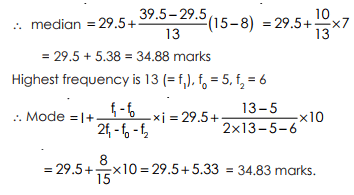(i) It can often be located by inspection.
(ii) It is not affected by extreme values. It is often a really typical value.
(iii) It is simple and precise. It is an actual item of the series except in a continuous series.
(iv) Mode can be determined graphically unlike Mean.

(i) It is unsuitable for algebraic treatment.
(ii) When the number of observations is small, the Mode may not exist, while the Mean and Median can be calculated.
(iii) The value of Mode is not based on each and every item of series.
(iv) It does not lead to the aggregate, if the Mode and the total number of items are given.

Empirical Relationship among Mean, Median and Mode
A distribution in which the values of Mean, Median and Mode coincide, is known symmetrical and if the above values are not equal, then the distribution is said asymmetrical or skewed. In a moderately skewed distribution, there is a relation amongst Mean, Median and Mode which is as follows :
Mean – Mode = 3 (Mean – Median)
If any two values are known, we can find the other

Example 40 : In a moderately asymmetrical distribution the mode and mean are 32.1 and 35.4 respectively. Calculate the Median. From the relation, we find
3 Median = 2 Mean + Mode
or 3 Median = 2 × 35.4 + 32.1 = 70.8 + 32.1 = 102.9
∴ Median = 34.3

The document Mode - Measures of Central Tendency, Business Mathematics & Statistics | Business Mathematics and Statistics - B Com is a part of the B Com Course Business Mathematics and Statistics.
All you need of B Com at this link: B Com

115 videos|142 docs

## FAQs on Mode - Measures of Central Tendency, Business Mathematics & Statistics - Business Mathematics and Statistics - B Com

 1. What is the mode in statistics?The mode in statistics refers to the value or values that appear most frequently in a dataset. It is a measure of central tendency and is used to describe the most common or typical value in a set of data.
 2. How is the mode calculated in statistics?To calculate the mode in statistics, you need to identify the value or values that occur with the highest frequency in a dataset. If there is only one value that appears most frequently, it is referred to as the mode. In cases where multiple values have the same highest frequency, the dataset is said to be multimodal, and all the values with the highest frequency are considered modes.
 3. When is the mode useful in business mathematics?The mode is useful in business mathematics when you want to identify the most common or popular value in a dataset. It can be used to analyze customer preferences, product sales, or any other data where you want to understand the most frequently occurring value. For example, a business may use the mode to determine the most popular product sold or the most common complaint received.
 4. Can a dataset have more than one mode?Yes, a dataset can have more than one mode. This occurs when multiple values have the same highest frequency in the dataset. For example, if a dataset contains the numbers 2, 4, 4, 6, 6, and 8, both 4 and 6 would be considered modes since they appear twice, which is more frequently than any other value in the dataset.
 5. How does the mode differ from the mean and median in statistics?The mode, mean, and median are all measures of central tendency in statistics, but they differ in how they represent the center of a dataset. - The mode represents the value(s) that appear most frequently. - The mean represents the average value of all the data points. - The median represents the middle value when the data is arranged in ascending or descending order. Each measure has its own use and interpretation, and the choice of which measure to use depends on the specific analysis and characteristics of the dataset.

115 videos|142 docsExplore Courses for B Com examSignup to see your scores go up within 7 days! Learn & Practice with 1000+ FREE Notes, Videos & Tests.
10M+ students study on EduRev
Track your progress, build streaks, highlight & save important lessons and more!
Related Searches

,

,

,

,

,

,

,

,

,

,

,

,

,

,

,

,

,

,

,

,

,

,

,

,

;